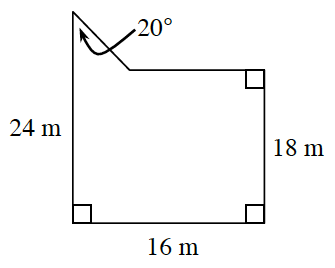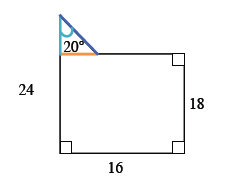### Home > CCG > Chapter 5 > Lesson 5.1.3 > Problem5-33

5-33.Find the area and the perimeter of the figure at right. Be sure to organize your work so you can explain your method later.

It will help if you break the shape into smaller, easier shapes.The blue side of the triangle is $24-18=6$.

Given the $20^\circ$ angle, and the length of the red side, what trig ratio can you use to determine the length of the blue triangle leg? Once you know the red and blue side, use the Pythagorean Theorem to find the length of the hypotenuse.

$\text{Perimeter}\approx78.21$ units (add length of all sides)
$\text{Area}\approx294.55\text{ units}^²$ (find area of triangle and rectangle)